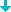List of Series/ Books

Total 12 Series/ Books
Number of Pages: 1
Sort By: | | |Most Recent

Maths Hub

Maths Hub, a set of 10 books from Pre-primer to class 8, is based exclusively on the latest curriculum prescribed by the council for the Indian Sch... Read More...

Mathematics Activity Manuals

These activity manuals have been designed to help students understand mathematical facts through a hands-on approach. Projects have been worked out... Read More...

Saraswati Mathematics (11 and 12)

Mathematics textbooks for classes 11 and 12 follow the latest core syllabus prescribed by the CBSE. These books have been developed keeping in mind th... Read More...

Mental Mathematics

This series has been designed to sharpen the mathematical skills of students. Students can improve their mental calculation abilities and learn to ... Read More...

Saraswati Mathematics (9 and 10)

This series for classes 9 and 10 follows the latest CBSE syllabus. It is based on the Continuous and Comprehensive Evaluation (CCE) scheme. Each co... Read More...

Math Magic

Math Magic Workbook is a maths practice series for classes 1 to 5 based on NCERT textbook. It provides plenty of interesting and systematic exercis... Read More...

Maths Quest

Maths Quest is a set of 10 books for pre-primer to class 8. This series has been conceived in such a manner that the students can learn and teachers c... Read More...

Destination Maths

Destination Maths is a series in which mathematical concepts are explained in an easy-to-understand manner. The concepts follow correct academic pr... Read More...

Me 'n' Mine Mathematics

In each book of this series, the emphasis is on interactive learning through activities and exercises. The concepts are taught with the help of varied... Read More...

Math Space

Math Space is a series of 5 books for classes 1 to 5 and aims to teach maths in an interesting and interactive way. These books comprise creative prac... Read More...

Saraswati Mathematics (6 to 8)

Saraswati Mathematics 6 to 8 is a series that attempts to make the learning of maths an enjoyable, fun-filled process. The books aim to lay a sound fo... Read More...
Scroll down to load more results
No More Results